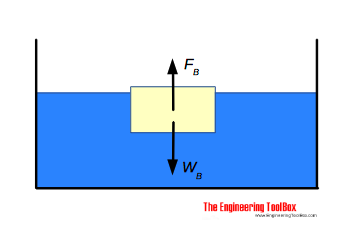Engineering ToolBox - Resources, Tools and Basic Information for Engineering and Design of Technical Applications!

# Archimedes' Law

## Forces acting on bodies submerged in fluids.Archimedes' principle states that:

"If a solid body floats or is submerged in a liquid - the liquid exerts an upward thrust force - a buoyant force - on the body equal to the gravitational force on the liquid displaced by the body."

The buoyant force can be expressed as

FB =  W

= V γ

= V ρ g                                     (1)

where

FB = buoyant force acting on submerged or floating body (N, lbf)

W = weight (gravity force) of displaced liquid (N, lbf)

V = volume of body below surface of liquid (m3, ft3)

γ = ρ g = specific weight of fluid (weight per unit volume) (N/m3, lbf/ft3)

ρ = density of fluid (kg/m3, slugs/ft3)

g = acceleration of gravity (9.81 m/s2, 32.174 ft/s2)

### Example - Density of a Body that floats in Water

A floating body is 95% submerged in water with density 1000 kg/m3.

For a floating body the buoyant force is equal to the weight of the water displaced by the body.

FB =  W

or

Vb ρb g = Vw ρw g

where

Vb = volume body (m3)

ρb = density of body (kg/m3)

Vw = volume of water (m3)

ρw  = density of water (kg/m3)

The equation can be transformed to

ρb = Vw ρw / Vb

Since 95% of the body is submerged

0.95 Vb = Vw

and the density of the body can be calculated as

ρb = 0.95 Vb (1000 kg/m3) / Vb

= 950 kg/m3

### Example - Buoyant force acting on a Brick submerged in Water

A standard brick with actual size 3 5/8 x 2 1/4 x 8 (inches) is submerged in water with density 1.940 slugs/ft3. The volume of the brick can be calculated

Vbrick = (3 5/8 in) (2 1/4 in) (8 in)

= 65.25 in3

The buoyant force acting on the brick is equal to the weight of the water displaced by the brick and can be calculated as

FB =  ((65.25 in3) / (1728 in/ft3)) (1.940 slugs/ft3) (32.174 ft/s2)

= 2.36 lbf

The weight or the gravity force acting on the brick - common red brick has specific gravity 1.75 - can be calculated to

WB = (2.36 lbf) 1.75

= 4.12 lbf

The resulting force acting on the brick can be calculated as

W(WB - FB) = (4.12 lbf) - (2.36 lbf)

= 1.76 lbf

## Related Topics

• ### Fluid Mechanics

The study of fluids - liquids and gases. Involving velocity, pressure, density and temperature as functions of space and time.

## Related Documents

• ### Hydrostatic Force acting on Submerged Surface

Calculate the thrust force acting on a submerged surface.
• ### Hydrostatic Pressure vs. Depth

Depth and hydrostatic pressure.

## Engineering ToolBox - SketchUp Extension - Online 3D modeling!

Add standard and customized parametric components - like flange beams, lumbers, piping, stairs and more - to your Sketchup model with the Engineering ToolBox - SketchUp Extension - enabled for use with older versions of the amazing SketchUp Make and the newer "up to date" SketchUp Pro . Add the Engineering ToolBox extension to your SketchUp Make/Pro from the Extension Warehouse !

We don't collect information from our users. More about

## Citation

• The Engineering ToolBox (2012). Archimedes' Law. [online] Available at: https://www.engineeringtoolbox.com/archimedes-principle-d_1845.html [Accessed Day Month Year].

Modify the access date according your visit.

9.19.12